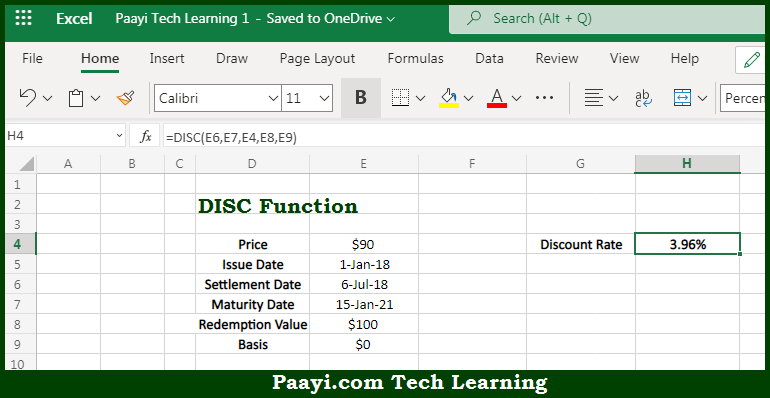# Learn How to Use Microsoft Excel DISC Function

Written by | 0 Comments | 480 Views

In this article, you will learn how to use the Microsoft Excel DISC function and its prime function in Microsoft Excel. You will also get to know the Microsoft Excel DISC function return value and syntax with the help of some examples.

Microsoft Excel DISC Function

The main purpose of the Microsoft Excel DISC function is to get the discount rate for security. That implies, with the help of the DISC function you can able to return the discount rate for the security. So, with the help of the DISC function, you can able to get the discount rate for the security.

Return Value of DISC Function

The return value will be the discount rate as a percentage.

Syntax of DISC Function

=DISC(settlement, maturity, pr, redemption, [basis])

Where the arguments:

• settlement: This is the settlement date of the security.
• maturity: This is the maturity date of the security.
• pr: This is the security price per \$100 face value.
• redemption: This is the security redemption value per \$100 face value.
• basis: This is the day count basis, defaults are 0 (optional).

## How to Use Microsoft Excel DISC Function?So we know that Microsoft Excel DISC function you can able to get get the discount rate for security. That implies, with the help of the DISC function you can able to return the discount rate for the security. So, with the help of the DISC function, you can able to get the discount rate for the security.